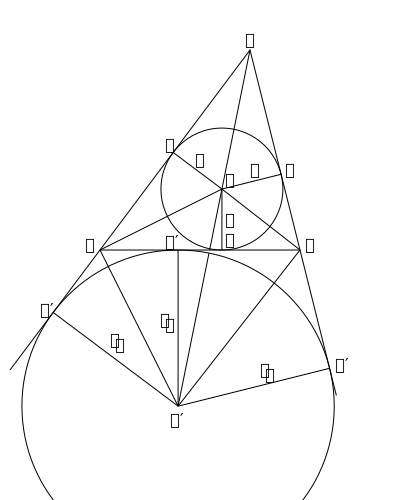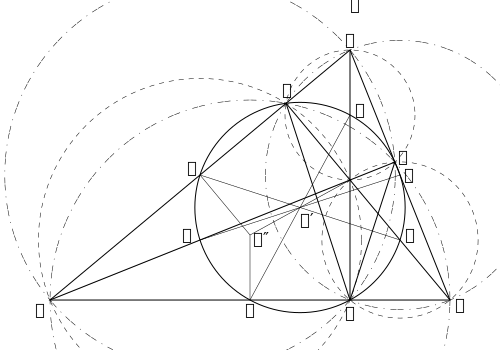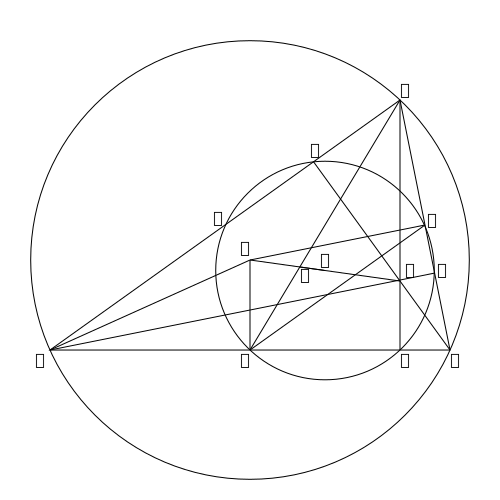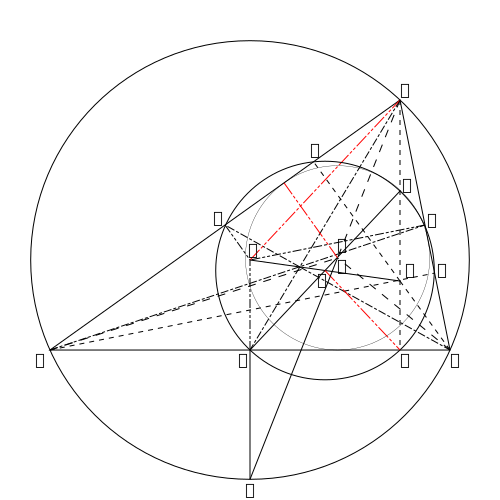output.to from Sideway
Draft for Information Only

# Content

`Elementary Geometry Miscellaneous Propositions  Proof  The Nine-Point Circle   Proof   Proof   Proof Sources and References`

# Elementary Geometry

## Miscellaneous Propositions

953If the inscribed circle of a triangle 𝐴𝐵𝐶 touches the sides 𝑎, 𝑏, 𝑐, in the points 𝐷, 𝐸, 𝐹; and if the escribed circle to the side 𝑎 touches 𝑎 and 𝑏, 𝑐 produced in 𝐷′, 𝐸′, 𝐹′; and if 𝑠=12(𝑎+𝑏+𝑐); then 𝐵𝐹′=𝐵𝐷′=𝐶𝐷′=𝑠−𝑐 and 𝐴𝐸′=𝐴𝐹′=𝑠 and similarly with respect to the other segments.

### Proof

The two tangents from any vertex to either circle being equal, it follows that 𝐶𝐷=𝑠−𝑐= half the perimeter of 𝐴𝐵𝐶, which is made up of three pairs of equal segments; therefore 𝐶𝐷=𝑠−𝑐 Also A𝐸′+A𝐹′=𝐴𝐶+𝐶𝐷′+𝐴𝐵+𝐵𝐷′ =2𝑠 therefore A𝐸′=A𝐹′=𝑠

### The Nine-Point Circle

954The Nine-point circle is the circle described through 𝐷, 𝐸, 𝐹, the feet of the erpendiculars on the sides of the triangle 𝐴𝐵𝐶. It also passes through the middle points of the sides of 𝐴𝐵𝐶 and the middle points of 𝑂𝐴, 𝑂𝐵, 𝑂𝐶; in all, through nine points.

#### Proof

Let the circle cut the sides of 𝐴𝐵𝐶 again in 𝐺, 𝐻, 𝐾; and 𝑂𝐴, 𝑂𝐵, 𝑂𝐶 in 𝐿, 𝑀, 𝑁. ∠𝐸𝑀𝐹=𝐸𝐷𝐹 (III. 21)=2𝑂𝐷𝐹 (952 Cor); therefore, since 𝑂𝐵 is the diameter of the circle circumscribing 𝑂𝐹𝐵𝐷 (III. 31), 𝑀 is the centre of that circle (III.20), and therefore bisects 𝑂𝐵.
Similarly 𝑂𝐶 and 𝑂𝑂 are bisected at 𝑁 and 𝐿.
Again, ∠𝑀𝐺𝐵=𝑀𝐸𝐷 (III.22)=𝑂𝐶𝐷, (III.21), by the circle circumscribing 𝑂𝐸𝐶𝐷. Therefore 𝑀𝐺 is parallel to 𝑂𝐶, and therefore bisects 𝐵𝐶. Similarly 𝐻 and 𝐾 bisect 𝐶𝐴 and 𝐴𝐵. 955The centre of the nine-point circle is the middle point of 𝑂𝑄, the line joining the orthocentre and the centre of the circumscribing circle of the triangle 𝐴𝐵𝐶.
For the centre of the 𝑁.𝑃. cirlce is the intersection of the perpendicular bisectors of the chords 𝐷𝐺, 𝐸𝐻, 𝐹𝐾, and these perpendiculars bisect 𝑂𝑄 in the same point 𝑁, by (VI.2). 956 The centroid of the triangle 𝐴𝐵𝐶 also lies on the line 𝑂𝑄 and divides it in 𝑅 so that 𝑂𝑅=2𝑅𝑄.

#### Proof

The triangles 𝑄𝐻𝐺, 𝑂𝐴𝐵 are similar, and 𝐴𝐵=2𝐻𝐺; therefore 𝐴𝑂=2𝐺𝑄; therefore 𝑂𝑅=2𝑅𝑄; and 𝐴𝑅=2𝑅𝐺; therefore 𝑅 is the centroid, and it divides 𝑂𝑄 as stated (951). 957 Hence the line joining the centres of the circumscribed and nine-point circles is divided harmonically in the ratio of 2∶1 by the centroid and the orthocentre of the triangle.
These two points are therefore centres of similitude of the circumscribed and nine-point circles; and any line drawn through either of the points is divided by the circumferences in the ratio of 2∶1. See (1037) 958 The lines 𝐷𝐸, 𝐸𝐹, 𝐹𝐷 intersect the sides of 𝐴𝐵𝐶 in the radical axis of the two circles.
For, if 𝐸𝐹 meets 𝐵𝐶 in 𝑃, then by the circle circumscribing 𝐵𝐶𝐸𝐹, 𝑃𝐸⋅𝑃𝐹=𝑃𝐶⋅𝑃𝐵; therefore (III.36) the tangents from 𝑃 to the circles are equal (985). 959The nine-point circle touches the inscribed and escribed circles of the triangl.

#### Proof

Let 𝑂 be the orthocentre, and 𝐼, 𝑄 the centres of the inscribed and circumscribed circles. Produce 𝐴𝐼 to bisect the arc 𝐵𝐶 in 𝑇. Bisect 𝐴𝑂 in 𝐿, and join 𝐺𝐿, cutting 𝐴𝑇 in 𝑆.
The 𝑁.𝑃. circle passes through 𝐺, 𝐷, 𝐿 (954), and 𝐷 is a right angle. Therefore 𝐺𝐿 is a diameter, and is therefore = 𝑅=𝑄𝐴 (957). Therefore 𝐺𝐿 and 𝑄𝐴 are parallel. But 𝑄𝐴=𝑄𝑇, therefore 𝐺𝑆=𝐺𝑇=𝐶𝑇sin𝐴2=2𝑅sin2𝐴2935,i Also 𝑆𝑇=2𝐺𝑆cos𝜃 (𝜃 being the angle 𝐺𝑆𝑇=𝐺𝑇𝑆).
𝑁 being the centre of the 𝑁.𝑃. circle, its radius=𝑁𝐺=12𝑅; and 𝑟 being the radius of the inscribed circle, it is required to shew that 𝑁𝐼=𝑁𝐺−𝑟 Now 𝑁𝐼2=𝑆𝑁2+𝑆𝐼2−2𝑆𝑁⋅𝑆𝐼cos𝜃 Substitute 𝑆𝑁=12𝑅−𝐺𝑆 𝑆𝐼=𝑇𝐼−𝑆𝑇=2𝑅sin𝐴2−2𝐺𝑆cos𝜃 and 𝐺𝑆=2𝑅sin212𝐴, to prove the proposition.
If 𝐽 be the centre of the escribd circle touching 𝐵𝐶, and 𝑟𝑎 its radius, it is shewn in a similar way that 𝑁𝐽=𝑁𝐺+𝑟𝑎.

## Sources and References

https://archive.org/details/synopsis-of-elementary-results-in-pure-and-applied-mathematics-pdfdrive

ID: 210900021 Last Updated: 9/21/2021 Revision: 0 Ref:References

1. Hilbert, D. (translated by Townsend E.J.), 1902, The Foundations of Geometry
2. Moore, E.H., 1902, On the projective axioms of geometry
3. Fitzpatrick R. (translated), Heiberg J.L. (Greek Text), Euclid (Author), 2008, Euclid's Elements of GeometryHome 5

Management

HBR 3

Information

Recreation

Culture

Chinese 1097

English 337

Computer

Hardware 154

Software

Application 207

Latex 35

Manim 203

Numeric 19

Programming

Web 285

Unicode 504

HTML 65

CSS 63

SVG 9

ASP.NET 240

OS 422

Python 64

Knowledge

Mathematics

Algebra 84

Geometry 32

Calculus 67

Engineering

Mechanical

Rigid Bodies

Statics 92

Dynamics 37

Control

Natural Sciences

Electric 27